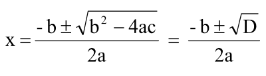# Introduction

## Definition

An expression of the form ax2 + bx + c, a ≠ 0, where a,b,c are constants, when equated to zero, represents a quadratic equation, with a,b,c as the coefficients.

The roots of the quadratic equation ax2 + bx + c = 0 are given by